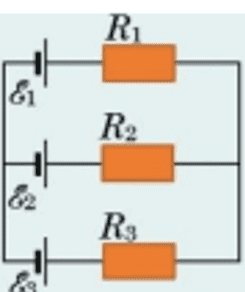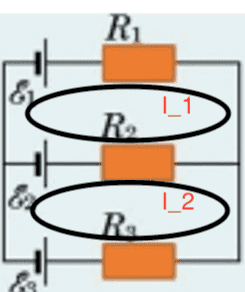# How to solve this chain with Kirchhoff's laws?

Oleksii
Homework Statement:
.
Relevant Equations:
.

Three current sources with EMF E1 = 11 V, E2 = 4 V and E3 = 6 V and three resistors with
resistance R1 = 5 Ohms, R2 = 10 Ohms and R3 = 2 Ohms are connected, as shown on picture below.
Determine the current strengths I in the resistors. Internal resistance sources neglect.How to solve this using Kirchhoff's laws? I understand that we have to make a system of 3 equations (because we have 3 variables), but the crux of the problem is that I don't know how to set them.

My attempt:
E1 - E2 = I1R1 - I2R2
E2 - E3 = I2R2 - I3R3
E1 - E2 - E3 = I1R1 - I2R2 - I3R3

Solve of this system:
I1 =11/5A
I2 = 2/5A
I3 = 3A

I took 12, 23, and 123 circles. But this answer is impossible because at least one of the currents has to be geative.

Last edited:

Staff Emeritus
Homework Helper
Gold Member
Please show us what you have attempted so far as per forum rules.

•topsquark
Homework Helper
Gold Member
I can see how you get the first two equations but not how you get the third. For this circuit, any choice of closed loop for applying the voltage rule can have only two emfs in it.
That said, the third equation should be a current conservation equation at one of the two nodes, ##I_1+I_2+I_3=0##.

Homework Helper
Gold Member
2022 Award
E1 - E2 = I1R1 - I2R2
E2 - E3 = I2R2 - I3R3
E1 - E2 - E3 = I1R1 - I2R2 - I3R3
Your first 2 equations are OK for the 2 inner loops.

The 3rd equation is completely wrong (and unnecessary – see @kuruman ’s Post #3).

The equation for the outer loop can not contain ##\mathscr E_2## and ##R_2## because these components are not part of the outer loop.

But if you want to check your understanding, post your corrected equation for the outer loop and we’ll check it for you.

Oleksii
Your first 2 equations are OK for the 2 inner loops.

The 3rd equation is completely wrong (and unnecessary – see @kuruman ’s Post #3).

The equation for the outer loop can not contain ##\mathscr E_2## and ##R_2## because these components are not part of the outer loop.

But if you want to check your understanding, post your corrected equation for the outer loop and we’ll check it for you.
But I am already not sure about first 2. E1 - I1R1 + I2R2 - E2 = 0, why I2R2 with plus, does it mean that second resistor create additional voltage and if so how it even possible?

Staff Emeritus
Homework Helper
Gold Member
But I am already not sure about first 2. E1 - I1R1 + I2R2 - E2 = 0, why I2R2 with plus, does it mean that second resistor create additional voltage and if so how it even possible?
The + or - depends on which direction you do the loop compared to how you defined the current. If you follow the loop around the same direction as the current, then voltage drops across the resistor. If you follow the loop against the current, voltage increases across the resistor.

Staff Emeritus
Homework Helper
But I am already not sure about first 2. E1 - I1R1 + I2R2 - E2 = 0, why I2R2 with plus, does it mean that second resistor create additional voltage and if so how it even possible?
To avoid mistakes, draw in the assumed direction for each current on the circuit and place a + where the current enters a resistor and a - at the opposite end, where the current leaves. Now, when writing the loop equations, if you go across a resistor from + to -, the term in the equation comes in with a minus sign. If you go from - to +, the term comes in with a plus sign.

The same method will also work when you start analyzing circuits with capacitors and inductors in a few weeks.

Staff Emeritus
Homework Helper
The 3rd equation is completely wrong (and unnecessary – see @kuruman ’s Post #3).
I'll add that if you were to write a corrected third equation, you should find it's simply some combination of the first two. In other words, mathematically, it's not an independent equation. Therefore, it's not useful if you already have the other two equations.

The third independent equation comes from apply the Kirchoff's current rule to one of the nodes, as @kuruman pointed out.

Oleksii
The + or - depends on which direction you do the loop compared to how you defined the current. If you follow the loop around the same direction as the current, then voltage drops across the resistor. If you follow the loop against the current, voltage increases across the resistor.
So okey. The first two eq are next:
E1 - E2 = I1R1 - I2R2
E2 - E3 = I2R2 = I3R3
Or one of them can be replaced by E1 - E3 = I1R1 - I3R3

So whats the third eq of system? How I can determine it?

Staff Emeritus
Homework Helper
Gold Member
So whats the third eq of system? How I can determine it?
Kirchhoff’s current law.

Oleksii
Kirchhoff’s current law.
Can you pls write it down? Because it's not clear for me. Is it always just I1 + I2 + I3 = 0 or I should at first choose some direction for current and only then use Kirchhoff’s current law based on pre-choosen direction?

Staff Emeritus
Homework Helper
Gold Member
Can you pls write it down? Because it's not clear for me. Is it always just I1 + I2 + I3 = 0 or I should at first choose some direction for current and only then use Kirchhoff’s current law based on pre-choosen direction?
KCL is based on the net current going into a node being zero. Based on your defined currents, they should be added if they go into the node and subtracted if they go out of a node.

Oleksii
KCL is based on the net current going into a node being zero. Based on your defined currents, they should be added if they go into the node and subtracted if they go out of a node.
How can I find direction of currents? I am understand form of KCL based on direction of currents but I can't understand how to find them. Biggest headeache that absolute value of currents in result depends on third eq.
And for me this situation looks like: I need to find direction of currents in order to solve system of equations but I have no idea how to find directions.

Staff Emeritus
Homework Helper
Just assume directions for each current. If you guessed the direction wrong for a current, you'll just get a negative answer.

Oleksii
Just assume directions for each current. If you guessed the direction wrong for a current, you'll just get a negative answer.
But what if I assumed directions(+I1, +I2, -I3 and +I1, -I2, -I3) and in both cases I get different non-negative results?

Staff Emeritus
Homework Helper
Gold Member
But what if I assumed directions(+I1, +I2, -I3 and +I1, -I2, -I3) and in both cases I get different non-negative results?
Again, you will get a result that tells you if the direction you guessed was correct or not in that a current will be negative if you guessed wrong. It is however absolutely crucial that you use the same current directional definitions in the KCL as you did in the KVL considerations.

Staff Emeritus
Homework Helper